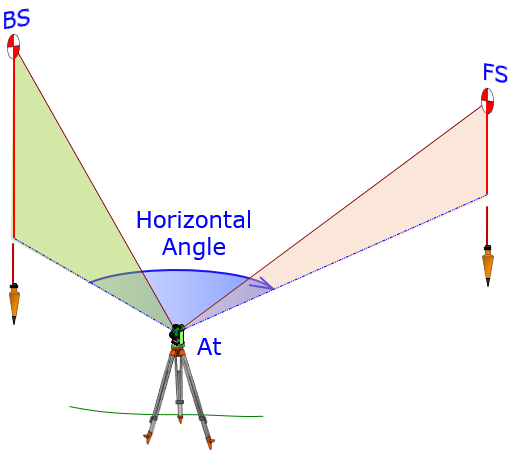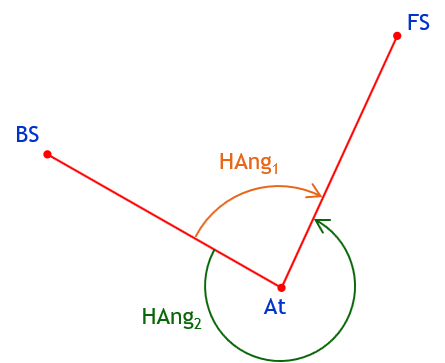## 1. Definition

A horizontal angle is measured perpendicular to gravity. Three points are involved:

• Backsight (BS)

• Foresight; FS

• At

The angle is between two vertical planes; one contains the BS and At points, the other contains the FS and At. The At point is generally occupied by a surveying instrument, Figure G-1.Figure G-1 Horizontal Angle

If we look down on the horizontal plane with the three points, Figure G-2, we see there are two horizontal angles between the vertical planes: HAng1 and HAng2.Figure G-2 Two Horizontal Angles

This causes an ambiguous situation unless we can specifically identify which angle we mean. Recall that the four parts of an angle are:

• Start

• Direction

• Magnitude

• End

HAng1 and HAng2 have the same start (BS) and end (FS) but different direction and magnitude. All four parts must be clearly defined. Merely including magnitude without a direction doesn’t resolve the ambiguity. For example, a 135° angle from BS to FS could still be interpreted two ways, Figure G-3.Figure G-3 Magnitude without Direction

Similarly, expressing a direction, to the right (clockwise) or left (counterclockwise), without a magnitude is also unclear, Figure G-4.Figure G-4 Direction without Magnitude

There are a few different ways to specify horizontal angles which help minimize confusing interpretations.